图像处理 增强对比度_传统图像处理方法知道哪些,图像对比度增强说一下 - CSDN

• 目标 在本教程中，您将学习如何： 访问像素值 用零初始化矩阵 了解cv :: saturate_cast的作用以及它有用的原因 获取有关像素转换的一些很酷的信息 在实际例子中提高图像的亮度 ...一般图像处理操作符是获取一...
目标

在本教程中，您将学习如何：

访问像素值
用零初始化矩阵
了解cv :: saturate_cast的作用以及它有用的原因
获取有关像素转换的一些很酷的信息
在实际例子中提高图像的亮度
理论

注意，以下解释属于Richard Szeliski 所着的Computer Vision：Algorithms and Applications一书

图像处理

一般图像处理操作符是获取一个或多个输入图像并产生输出图像的功能。
图像变换可以看作：
点运算（像素变换）-
Point operators (pixel transforms)
邻域操作
Neighborhood (area-based) operators

像素转换

在这种图像处理变换中，每个输出像素的值仅取决于相应的输入像素值。
这种算子的例子包括亮度和对比度调整以及颜色校正和变换。
亮度和对比度调整

两个常用的点过程是乘法和加法与常量：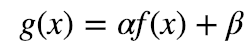参数α>0和β通常称为增益和偏差参数（the gain and bias parameters），这些参数分别控制对比度和亮度。

假设f(x)作为源图像像素，g（x）作为输出图像像素，上述表达式可以改写为：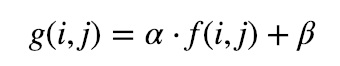其中，i和j表示像素位于第i行和第j列。

Coding
C ++

完整代码：
/**
* @file BasicLinearTransforms.cpp
* @brief Simple program to change contrast and brightness
* @author OpenCV team
*/

#include "opencv2/imgcodecs.hpp"
#include "opencv2/highgui.hpp"
#include <iostream>

// we're NOT "using namespace std;" here, to avoid collisions between the beta variable and std::beta in c++17
using std::cin;
using std::cout;
using std::endl;
using namespace cv;

/**
* @function main
* @brief Main function
*/
int main( int argc, char** argv )
{
/// Read image given by user
CommandLineParser parser( argc, argv, "{@input | ../data/lena.jpg | input image}" );
Mat image = imread( parser.get<String>( "@input" ) );
if( image.empty() )
{
cout << "Could not open or find the image!\n" << endl;
cout << "Usage: " << argv << " <Input image>" << endl;
return -1;
}

//! [basic-linear-transform-output]
Mat new_image = Mat::zeros( image.size(), image.type() );
//! [basic-linear-transform-output]

//! [basic-linear-transform-parameters]
double alpha = 1.0; /*< Simple contrast control */
int beta = 0;       /*< Simple brightness control */

/// Initialize values
cout << " Basic Linear Transforms " << endl;
cout << "-------------------------" << endl;
cout << "* Enter the alpha value [1.0-3.0]: "; cin >> alpha;
cout << "* Enter the beta value [0-100]: ";    cin >> beta;
//! [basic-linear-transform-parameters]

/// Do the operation new_image(i,j) = alpha*image(i,j) + beta
/// Instead of these 'for' loops we could have used simply:
/// image.convertTo(new_image, -1, alpha, beta);
/// but we wanted to show you how to access the pixels :)
//! [basic-linear-transform-operation]
for( int y = 0; y < image.rows; y++ ) {
for( int x = 0; x < image.cols; x++ ) {
for( int c = 0; c < image.channels(); c++ ) {
new_image.at<Vec3b>(y,x)[c] =
saturate_cast<uchar>( alpha*image.at<Vec3b>(y,x)[c] + beta );
}
}
}
//! [basic-linear-transform-operation]

//! [basic-linear-transform-display]
/// Show stuff
imshow("Original Image", image);
imshow("New Image", new_image);

/// Wait until user press some key
waitKey();
//! [basic-linear-transform-display]
return 0;
}

说明

  CommandLineParser parser( argc, argv, "{@input | ../data/lena.jpg | input image}" );
Mat image = imread( parser.get<String>( "@input" ) );
if( image.empty() )
{
cout << "Could not open or find the image!\n" << endl;
cout << "Usage: " << argv << " <Input image>" << endl;
return -1;
}

现在，由于我们将对此图像进行一些转换，因此我们需要一个新的Mat对象来存储它。此外，我们希望它具有以下功能：

初始像素值等于零
与原始图像的大小和类型相同

 Mat new_image = Mat::zeros( image.size(), image.type() );
// the same size and type of Mat img


我们观察到cv :: Mat :: zeros返回一个基于image.size（）和image.type（）的Matlab样式的零初始值设定项

我们现在问α的值和β 由用户输入：
 double alpha = 1.0; /*< Simple contrast control */
int beta = 0;       /*< Simple brightness control */
cout << " Basic Linear Transforms " << endl;
cout << "-------------------------" << endl;
cout << "* Enter the alpha value [1.0-3.0]: "; cin >> alpha;
cout << "* Enter the beta value [0-100]: ";    cin >> beta;


现在，执行操作g（i，j）= α · f（i，j）+ b我们将访问图像中的每个像素。由于我们使用BGR图像进行操作，因此每个像素（B，G和R）将有三个值，因此我们也将单独访问它们。
代码：
 for( int y = 0; y < image.rows; y++ ) {
for( int x = 0; x < image.cols; x++ ) {
for( int c = 0; c < image.channels(); c++ ) {
new_image.at<Vec3b>(y,x)[c] =
saturate_cast<uchar>( alpha*image.at<Vec3b>(y,x)[c] + beta );
}
}
}


请注意以下内容（仅限C ++代码）：

要访问图像中的每个像素，我们使用以下语法：image.at <Vec3b>（y，x）[c]其中y是行，x是列，c是R，G或B（0,1或2）。
由于操作α＆CenterDot;＆p（i，j）+ b可以给出超出范围的值或不是整数（如果α是浮动），我们使用cv :: saturate_cast来确保值是有效的。
最后，我们创建窗口并以通常的方式显示图像。

    imshow("Original Image", image);
imshow("New Image", new_image);
waitKey();


注意

我们可以简单地使用此命令，而不是使用for循环来访问每个像素：

image.convertTo（new_image，-1，alpha，beta）;


其中cv :: Mat :: convertTo将有效地执行* new_image = a * image + beta *。但是，我们想向您展示如何访问每个像素。在任何情况下，两种方法都给出相同的结果，但convertTo更优化，工作速度更快。

结果

运行我们的代码并使用α=2.2和β= 50
\$ ./BasicLinearTransforms lena.jpg

基本线性变换

-------------------------

*输入alpha值[1.0-3.0]：2.2

*输入beta值[0-100]：50

我们得到这个：例子

在本段中，我们将通过调整图像的亮度和对比度来实践我们所学到的校正曝光不足图像的方法。我们还将看到另一种技术来校正称为伽马校正图像亮度。

亮度和对比度调整

增加（/减少）β将为每个像素添加（/减去）一个常量值。像素值在[0; 255]范围将饱和（即，高于（/小于）255（/ 0）的像素值将被截止到255（/ 0））。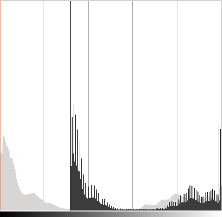原始图像的直方图，当Gimp中亮度= 80时为深灰色

直方图针对每个颜色级别表示具有该颜色级别的像素的数量。暗图像将具有许多具有低颜色值的像素，因此直方图将在其左侧部分呈现峰值。当添加恒定偏置时，直方图向右移动，因为我们已经为所有像素添加了恒定偏置。

在一个参数将修改级别的传播方式。如果α<1，颜色等级将被压缩，结果将是对比度较低的图像。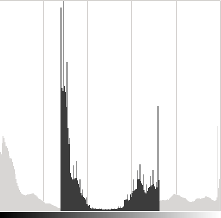原始图像的直方图，当在Gimp中对比度<0时为深灰色

请注意，这些直方图是使用Gimp软件中的亮度 - 对比度工具获得的。亮度工具应与β相同偏差参数但对比工具似乎与α不同 输出范围似乎以Gimp为中心的增益（正如您在前面的直方图中所注意到的那样）。

β可能会发生偏差会改善亮度，但同时图像会出现轻微的面纱，因为对比度会降低。该α 增益可以用来减少这种效果，但由于饱和，我们将失去原始明亮区域的一些细节。

伽玛校正

通过在输入值和映射的输出值之间使用非线性变换，Gamma校正可用于校正图像的亮度：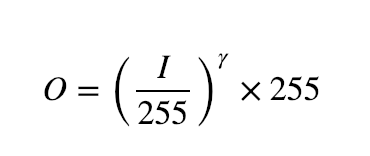由于此关系是非线性的，因此对于所有像素，效果将不相同，并且将取决于它们的原始值。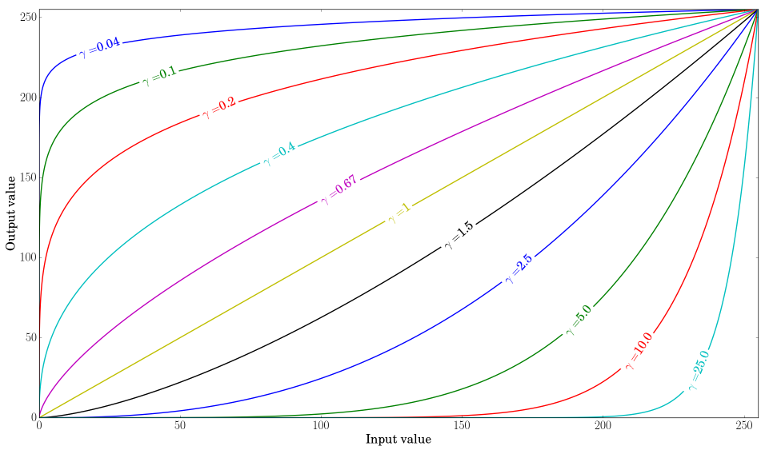绘制不同的伽玛值，当γ< 1中，原始的暗区将是明亮，直方图将被向右移位，而这将是与相对的γ> 1。

纠正曝光不足的图像

以下图像已更正为：α=1.3和β= 40。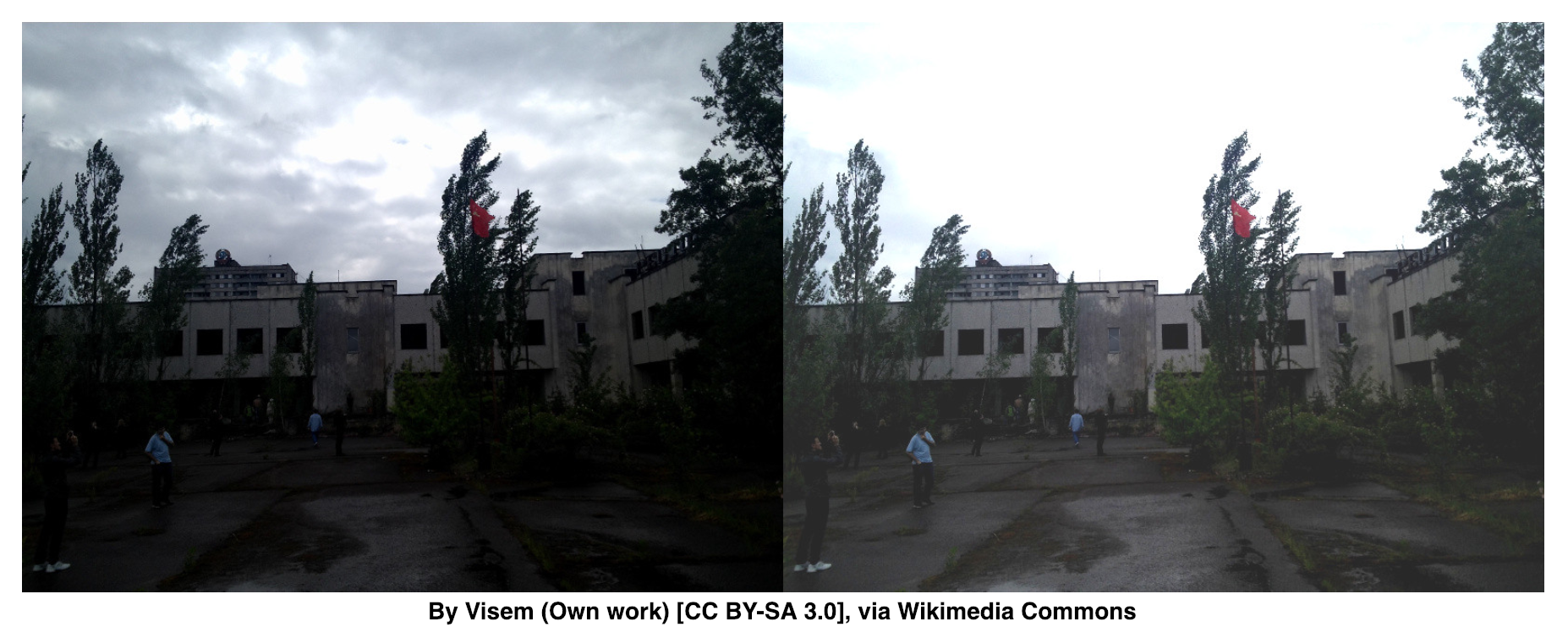整体亮度已得到改善，但您可以注意到，经过对比度调整之后，暗部细节得到了明显改善，与此同时，高亮度部分的云已经过饱和，使得天空颜色失真。

以下图像已用：γ校正= 0.4。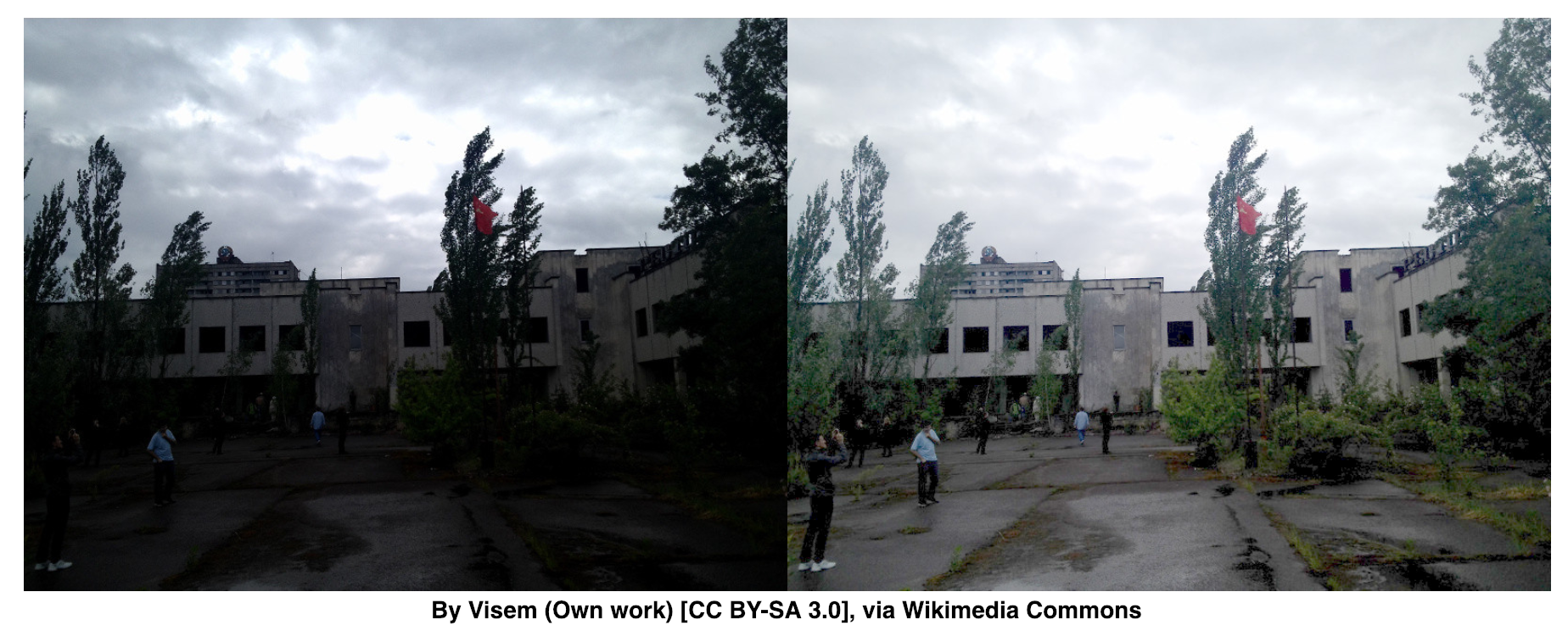伽马校正应该倾向于增加图像的动态对比度，因为映射是非线性的并且不存在如先前方法中那样的数值饱和。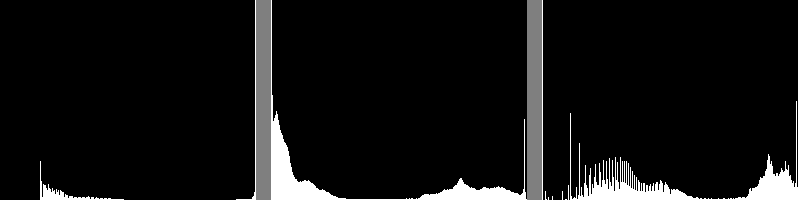左：alpha，beta校正后的直方图;  中：原始图像的直方图; 右：伽马校正后的直方图

上图比较了三幅图像的直方图（三个直方图之间的y范围不同）。您可以注意到，大多数像素值位于原始图像直方图的下半部分。在α之后，b校正，由于饱和以及右移，我们可以观察到255处的大峰值。在伽马校正之后，直方图向右移动但是暗区域中的像素比亮区域中的像素更加偏移（参见伽马曲线图）。

伽马变换代码段：

C++

 Mat lookUpTable(1, 256, CV_8U);
uchar* p = lookUpTable.ptr();
for( int i = 0; i < 256; ++i)
p[i] = saturate_cast<uchar>(pow(i / 255.0, gamma_) * 255.0);
Mat res = img.clone();
LUT(img, lookUpTable, res);

完整代码：

#include <iostream>
#include "opencv2/imgcodecs.hpp"
#include "opencv2/highgui.hpp"

// we're NOT "using namespace std;" here, to avoid collisions between the beta variable and std::beta in c++17
using std::cout;
using std::endl;
using namespace cv;

namespace
{
/** Global Variables */
int alpha = 100;
int beta = 100;
int gamma_cor = 100;
Mat img_original, img_corrected, img_gamma_corrected;

void basicLinearTransform(const Mat &img, const double alpha_, const int beta_)
{
Mat res;
img.convertTo(res, -1, alpha_, beta_);

hconcat(img, res, img_corrected);
}

void gammaCorrection(const Mat &img, const double gamma_)
{
CV_Assert(gamma_ >= 0);
//! [changing-contrast-brightness-gamma-correction]
Mat lookUpTable(1, 256, CV_8U);
uchar* p = lookUpTable.ptr();
for( int i = 0; i < 256; ++i)
p[i] = saturate_cast<uchar>(pow(i / 255.0, gamma_) * 255.0);

Mat res = img.clone();
LUT(img, lookUpTable, res);
//! [changing-contrast-brightness-gamma-correction]

hconcat(img, res, img_gamma_corrected);
imshow("Gamma correction", img_gamma_corrected);
}

void on_linear_transform_alpha_trackbar(int, void *)
{
double alpha_value = alpha / 100.0;
int beta_value = beta - 100;
basicLinearTransform(img_original, alpha_value, beta_value);
}

void on_linear_transform_beta_trackbar(int, void *)
{
double alpha_value = alpha / 100.0;
int beta_value = beta - 100;
basicLinearTransform(img_original, alpha_value, beta_value);
}

void on_gamma_correction_trackbar(int, void *)
{
double gamma_value = gamma_cor / 100.0;
gammaCorrection(img_original, gamma_value);
}
}

int main( int argc, char** argv )
{
CommandLineParser parser( argc, argv, "{@input | ../data/lena.jpg | input image}" );
img_original = imread( parser.get<String>( "@input" ) );
if( img_original.empty() )
{
cout << "Could not open or find the image!\n" << endl;
cout << "Usage: " << argv << " <Input image>" << endl;
return -1;
}

img_corrected = Mat(img_original.rows, img_original.cols*2, img_original.type());
img_gamma_corrected = Mat(img_original.rows, img_original.cols*2, img_original.type());

hconcat(img_original, img_original, img_corrected);
hconcat(img_original, img_original, img_gamma_corrected);

namedWindow("Gamma correction");

createTrackbar("Alpha gain (contrast)", "Brightness and contrast adjustments", &alpha, 500, on_linear_transform_alpha_trackbar);
createTrackbar("Beta bias (brightness)", "Brightness and contrast adjustments", &beta, 200, on_linear_transform_beta_trackbar);
createTrackbar("Gamma correction", "Gamma correction", &gamma_cor, 200, on_gamma_correction_trackbar);

on_linear_transform_alpha_trackbar(0, 0);
on_gamma_correction_trackbar(0, 0);

waitKey();

imwrite("linear_transform_correction.png", img_corrected);
imwrite("gamma_correction.png", img_gamma_corrected);

return 0;
}


Python

lookUpTable = np.empty((1,256), np.uint8)
for i in range(256):
lookUpTable[0,i] = np.clip(pow(i / 255.0, gamma) * 255.0, 0, 255)
res = cv.LUT(img_original, lookUpTable)

完整代码：

from __future__ import print_function
from __future__ import division
import cv2 as cv
import numpy as np
import argparse

alpha = 1.0
alpha_max = 500
beta = 0
beta_max = 200
gamma = 1.0
gamma_max = 200

def basicLinearTransform():
res = cv.convertScaleAbs(img_original, alpha=alpha, beta=beta)
img_corrected = cv.hconcat([img_original, res])

def gammaCorrection():
## [changing-contrast-brightness-gamma-correction]
lookUpTable = np.empty((1,256), np.uint8)
for i in range(256):
lookUpTable[0,i] = np.clip(pow(i / 255.0, gamma) * 255.0, 0, 255)

res = cv.LUT(img_original, lookUpTable)
## [changing-contrast-brightness-gamma-correction]

img_gamma_corrected = cv.hconcat([img_original, res]);
cv.imshow("Gamma correction", img_gamma_corrected);

def on_linear_transform_alpha_trackbar(val):
global alpha
alpha = val / 100
basicLinearTransform()

def on_linear_transform_beta_trackbar(val):
global beta
beta = val - 100
basicLinearTransform()

def on_gamma_correction_trackbar(val):
global gamma
gamma = val / 100
gammaCorrection()

parser = argparse.ArgumentParser(description='Code for Changing the contrast and brightness of an image! tutorial.')
parser.add_argument('--input', help='Path to input image.', default='lena.jpg')
args = parser.parse_args()

if img_original is None:
print('Could not open or find the image: ', args.input)
exit(0)

img_corrected = np.empty((img_original.shape, img_original.shape*2, img_original.shape), img_original.dtype)
img_gamma_corrected = np.empty((img_original.shape, img_original.shape*2, img_original.shape), img_original.dtype)

img_corrected = cv.hconcat([img_original, img_original])
img_gamma_corrected = cv.hconcat([img_original, img_original])

cv.namedWindow('Gamma correction')

alpha_init = int(alpha *100)
cv.createTrackbar('Alpha gain (contrast)', 'Brightness and contrast adjustments', alpha_init, alpha_max, on_linear_transform_alpha_trackbar)
beta_init = beta + 100
cv.createTrackbar('Beta bias (brightness)', 'Brightness and contrast adjustments', beta_init, beta_max, on_linear_transform_beta_trackbar)
gamma_init = int(gamma * 100)
cv.createTrackbar('Gamma correction', 'Gamma correction', gamma_init, gamma_max, on_gamma_correction_trackbar)

on_linear_transform_alpha_trackbar(alpha_init)
on_gamma_correction_trackbar(gamma_init)

cv.waitKey()

查找线性表用于提高计算性能，因为只需要计算一次256个值。

展开全文数字图像处理 亮度调整 图像增强
• 对比度增强一直是图像处理中的一个恒久话题，一般来说都是基于直方图的，比如直方图均衡化。冈萨雷斯的书里面对这个话题讲的比较透彻。 关于图像增强必须清楚的基本概念 1.图像增强的目的： 1）改善图像的视觉...
Contrast Enhancement：
对比度增强一直是图像处理中的一个恒久话题，一般来说都是基于直方图的，比如直方图均衡化。冈萨雷斯的书里面对这个话题讲的比较透彻。
关于图像增强必须清楚的基本概念
1.图像增强的目的：
1）改善图像的视觉效果，
2）转换为更适合于人或机器分析处理的形式
3）突出对人或机器分析有意义的信息
4）抑制无用信息，提高图像的使用价值
5）增强后的图像并不一定保真
2，图像增强的方法分类：
1）从处理对象分类：灰度图像，（伪）彩色图像
2）从处理策略分类：全局处理，局部处理（ROI ROI，Region of Interest Interest）
3）从处理方法分类：空间域（点域运算，即灰度变换；邻域方法，即空域滤波），频域方法
4）从处理目的分类：图像锐化，平滑去噪，灰度调整（对比度增强）
3.图像增强的方法：
（1）. 基于直方图均衡化的图像增强（Histogram Equalization）
a. 基本思想
对于图像中的灰度点做映射，使得整体图像的灰度大致符合均匀分布。增强图像的对比度。

对于灰度值连续的情况，使用灰度的累积分布函数CDF做转换函数，可以使得输出图像的灰度符合均匀分布。
对于灰度值不连续的情况，存在舍入误差，得到的灰度分布大致符合均匀分布。
直观地理解，如果某一个灰度范围（如200-201）的像素点很少，那么它的概率密度值就会很小，所以CDF在200-201附近的增长变化就会很小；反之，如果某一个灰度范围（如100-101）的像素点很多，CDF在100-101附近的增长变化会很大。总体来看，以灰度为横轴，CDF为纵轴画曲线。这种向上凸的曲线，很像gamma变换: s=crγs=crγ中γ<1γ<1的情形。将灰度集中的部分拉伸，而将灰度不集中的部分压缩，达到提高对比度的效果。
直方图均衡可以看做自适应的gamma变换或者分段变换。前者的优势在于，不需要指定任何参数，所有运算都是基于图像本身的。

b. 算法
step1：根据图像灰度计算灰度概率密度函数PDF
step2：计算累积概率分布函数CDF
step3：将CDF归一化到原图灰度取值范围，如[0,255]。
step4：之后CDF四舍五入取整，得到灰度转换函数sk=T(rk)
step5：将CDF作为转换函数，将灰度为rk的点转换为sk灰度
直方图均衡化是通过调整图像的灰阶分布，使得在0~255灰阶上的分布更加均衡，提高了图像的对比度，达到改善图像主观视觉效果的目的。对比度较低的图像适合使用直方图均衡化方法来增强图像细节。
彩色图像的直方图均衡化实现：
#include <opencv2/opencv.hpp>
#include <iostream>

using namespace cv;
using namespace std;

int main(int argc, char *argv[])
{
if (image.empty())
{
cout << "could not load images.." << endl;
return -1;
}
imshow("src", image);
Mat imageRGB;
split(image, imageRGB);
for (int i = 0; i < 3; i++)
{
equalizeHist(imageRGB[i], imageRGB[i]);
}
merge(imageRGB, 3, image);
imshow("equalizeHist", image);
waitKey(0);
return 0;
}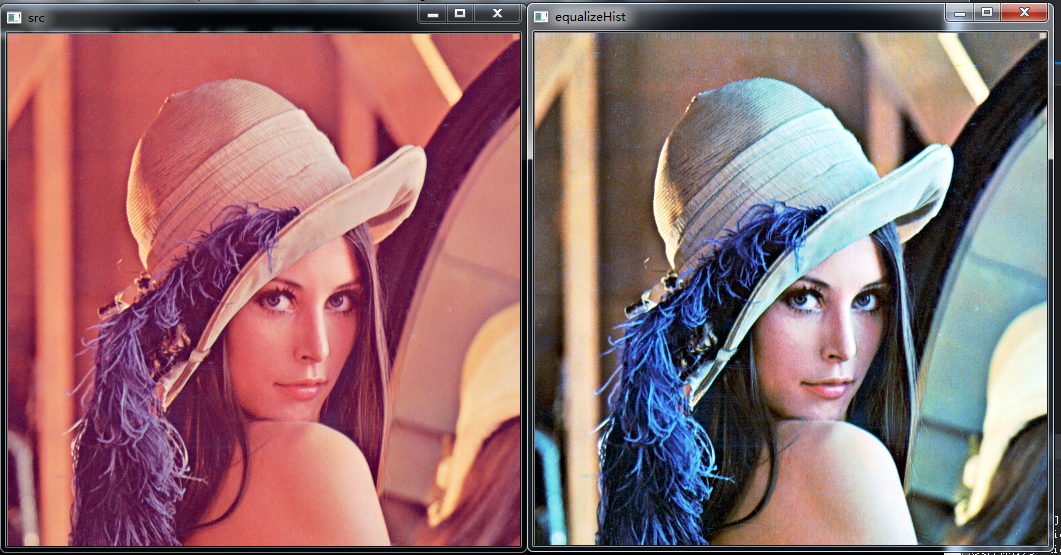（2）. 基于拉普拉斯算子的图像增强
使用中心为5的8邻域拉普拉斯算子与图像卷积可以达到锐化增强图像的目的，拉普拉斯算子如下图所示：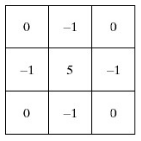#include<opencv2/opencv.hpp>
#include<iostream>

using namespace cv;
using namespace std;
int main(int argc, char *argv[])
{
if (image.empty())
{
cout << "could not load images..." << endl;
return -1;
}
imshow("原图像", image);
Mat imageEnhance;
Mat kernel = (Mat_<float>(3, 3) << 0, -1, 0, 0, 5, 0, 0, -1, 0);
filter2D(image, imageEnhance, CV_8UC3, kernel);
imshow("拉普拉斯算子图像增强效果", imageEnhance);
waitKey(0);
return 0;
}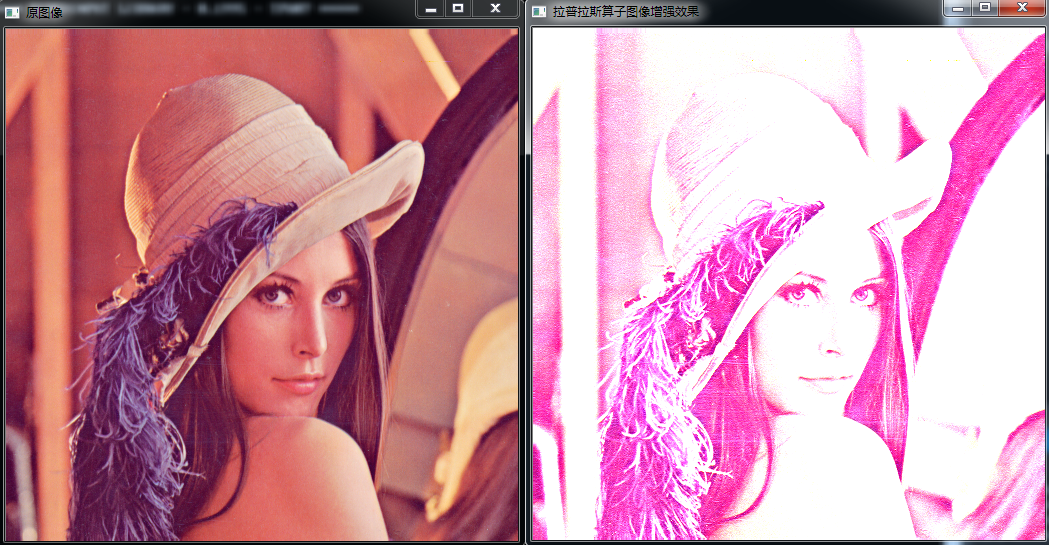（3）.基于对数Log变换的图像增强
对数变换可以将图像的低灰度值部分扩展，显示出低灰度部分更多的细节，将其高灰度值部分压缩，减少高灰度值部分的细节，从而达到强调图像低灰度部分的目的。变换方法：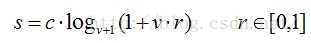对数变换对图像低灰度部分细节增强的功能过可以从对数图上直观理解：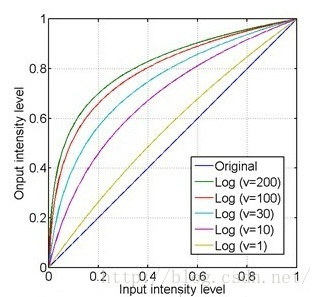x轴的0.4大约对应了y轴的0.8，即原图上00.4的低灰度部分经过对数运算后扩展到00.8的部分，而整个0.41的高灰度部分被投影到只有0.81的区间，这样就达到了扩展和增强低灰度部分，压缩高灰度部分的值的功能。
从上图还可以看到，对于不同的底数，底数越大，对低灰度部分的扩展就越强，对高灰度部分的压缩也就越强。
#include <opencv2/opencv.hpp>
#include<iostream>

using namespace std;
using namespace cv;

int main(int argc, char *argv[])
{
Mat imageLog(image.size(), CV_32FC3);
for (int i = 0; i < image.rows; i++)
{
for (int j = 0; j < image.cols; j++)
{
imageLog.at<Vec3f>(i, j) = log(1 + image.at<Vec3b>(i, j));
imageLog.at<Vec3f>(i, j) = log(1 + image.at<Vec3b>(i, j));
imageLog.at<Vec3f>(i, j) = log(1 + image.at<Vec3b>(i, j));
}
}
//归一化到0~255
normalize(imageLog, imageLog, 0, 255, CV_MINMAX);
//转换成8bit图像显示
convertScaleAbs(imageLog, imageLog);
imshow("src", image);
imshow("基于对数Log变换的图像增强", imageLog);
waitKey();
return 0;
}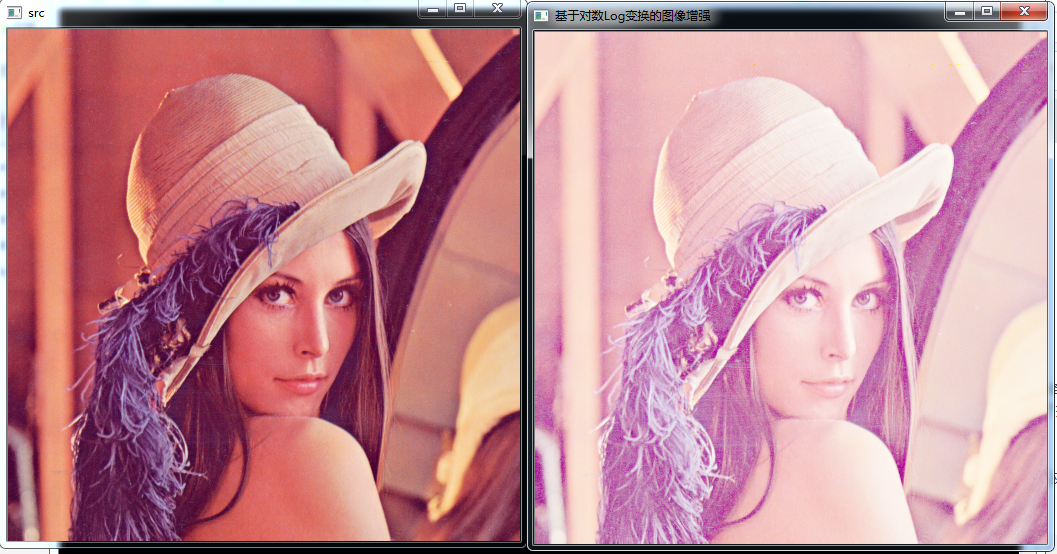（4）. 基于伽马（Gamma）变换的图像增强
伽马变换主要用于图像的校正，将灰度过高或者灰度过低的图片进行修正，增强对比度。变换公式就是对原图像上每一个像素值做乘积运算：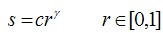伽马变换对图像的修正作用其实就是通过增强低灰度或高灰度的细节实现的，从伽马曲线可以直观理解：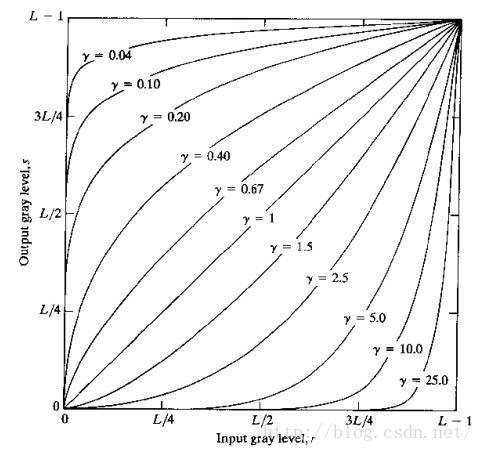γ值以1为分界，值越小，对图像低灰度部分的扩展作用就越强，值越大，对图像高灰度部分的扩展作用就越强，通过不同的γ值，就可以达到增强低灰度或高灰度部分细节的作用。
伽马变换对于图像对比度偏低，并且整体亮度值偏高（对于于相机过曝）情况下的图像增强效果明显。
#include <opencv2/opencv.hpp>
#include <iostream>

using namespace std;
using namespace cv;

int main(int argc, char *argv[])
{
Mat imageGamma(image.size(), CV_32FC3);
for (int i = 0; i < image.rows; i++)
{
for (int j = 0; j < image.cols; j++)
{
imageGamma.at<Vec3f>(i, j) = (image.at<Vec3b>(i, j))*(image.at<Vec3b>(i, j))*(image.at<Vec3b>(i, j));
imageGamma.at<Vec3f>(i, j) = (image.at<Vec3b>(i, j))*(image.at<Vec3b>(i, j))*(image.at<Vec3b>(i, j));
imageGamma.at<Vec3f>(i, j) = (image.at<Vec3b>(i, j))*(image.at<Vec3b>(i, j))*(image.at<Vec3b>(i, j));
}
}
//归一化到0~255
normalize(imageGamma, imageGamma, 0, 255, CV_MINMAX);
//转换成8bit图像显示
convertScaleAbs(imageGamma, imageGamma);
imshow("原图", image);
imshow("伽马变换图像增强效果", imageGamma);
waitKey();
return 0;
}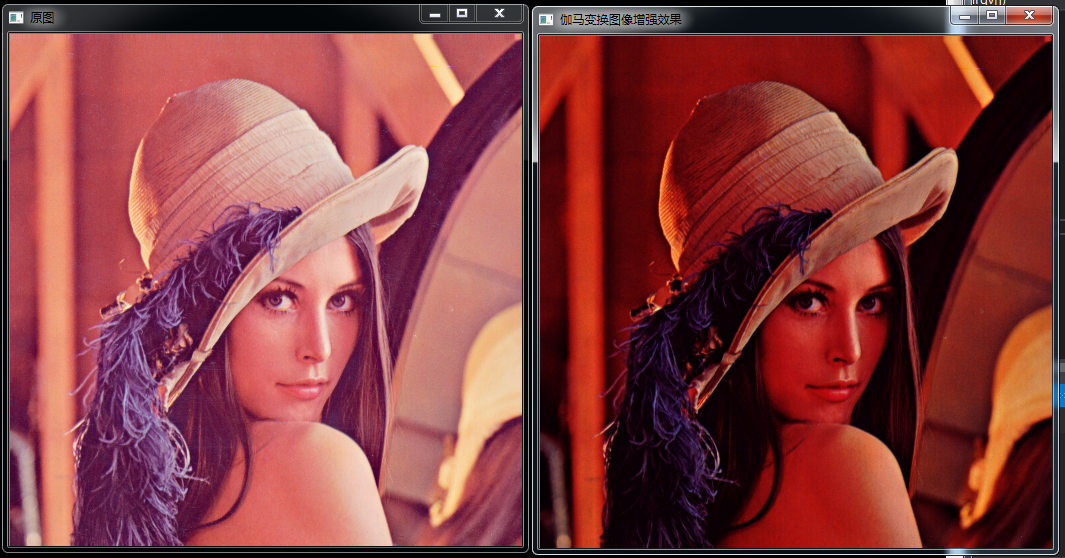展开全文• 关于图像增强必须清楚的基本概念 1.图像增强的目的： 1）改善图像的视觉效果， 2）转换为更适合于人或机器分析处理的形式 3）突出对人或机器分析有意义的信息 4）抑制无用信息，提高图像的使用价值 5）...
关于图像增强必须清楚的基本概念

1.图像增强的目的：

1）改善图像的视觉效果，
2）转换为更适合于人或机器分析处理的形式
3）突出对人或机器分析有意义的信息
4）抑制无用信息，提高图像的使用价值
5）增强后的图像并不一定保真

2，图像增强的方法分类：

1）从处理对象分类：灰度图像，（伪）彩色图像
2）从处理策略分类：全局处理，局部处理（ROI ROI，Region of Interest Interest）
3）从处理方法分类：空间域（点域运算，即灰度变换；邻域方法，即空域滤波），频域方法
4）从处理目的分类：图像锐化，平滑去噪，灰度调整（对比度增强）

3，图像增强的方法之对比度增强

1）灰度变换法

线性变换（已实现）
对数变换（已实现）
指数变换（已实现）

2）直方图调整法
直方图均衡化（已实现）
直方图匹配（未实现）

一，直方图均衡化

直方图均衡化的英文名称是Histogram Equalization.
图像对比度增强的方法可以分成两类:一类是直接对比度增强方法;另一类是间接对比度增强方法。直方图拉伸和直方图均衡化是两种最常见的间接对比度增强方法。直方图拉伸是通过对比度拉伸对直方图进行调整，从而“扩大”前景和背景灰度的差别，以达到增强对比度的目的，这种方法可以利用线性或非线性的方法来实现;直方图均衡化则通过使用累积函数对灰度值进行“调整”以实现对比度的增强。
直方图均衡化处理的“中心思想”是把原始图像的灰度直方图从比较集中的某个灰度区间变成在全部灰度范围内的均匀分布。直方图均衡化就是对图像进行非线性拉伸，重新分配图像像素值，使一定灰度范围内的像素数量大致相同。直方图均衡化就是把给定图像的直方图分布改变成“均匀”分布直方图分布。
缺点：
1）变换后图像的灰度级减少，某些细节消失；
2）某些图像，如直方图有高峰，经处理后对比度不自然的过分增强。
直方图均衡化是图像处理领域中利用图像直方图对对比度进行调整的方法。
这种方法通常用来增加许多图像的局部对比度，尤其是当图像的有用数据的对比度相当接近的时候。通过这种方法，亮度可以更好地在直方图上分布。这样就可以用于增强局部的对比度而不影响整体的对比度，直方图均衡化通过有效地扩展常用的亮度来实现这种功能。
这种方法对于背景和前景都太亮或者太暗的图像非常有用，这种方法尤其是可以带来X光图像中更好的骨骼结构显示以及曝光过度或者曝光不足照片中更好的细节。这种方法的一个主要优势是它是一个相当直观的技术并且是可逆操作，如果已知均衡化函数，那么就可以恢复原始的直方图，并且计算量也不大。这种方法的一个缺点是它对处理的数据不加选择，它可能会增加背景杂讯的对比度并且降低有用信号的对比度。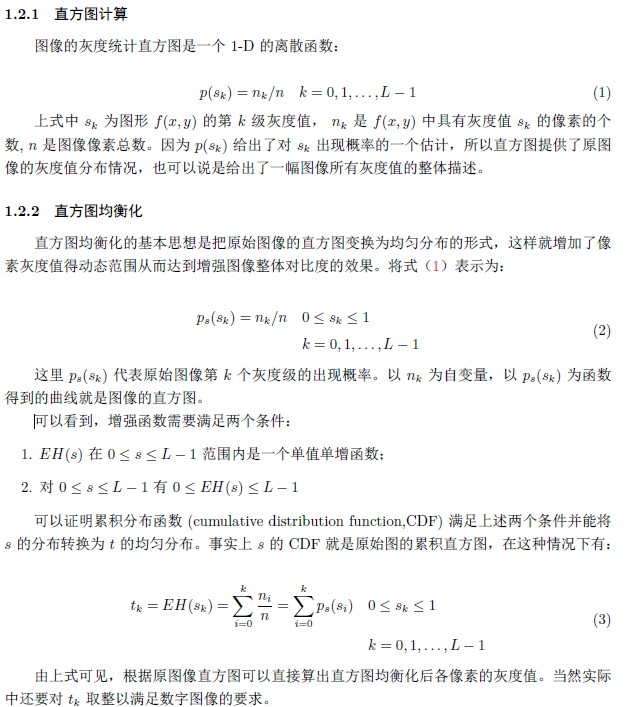关于编程实现，同样是不调用matlab库函数，自己编程实现。这样可以更深刻地理解直方图均衡化技术，提高编程能力。

实现代码（matlab）：

[cpp] view
plain copy

print?

clc;
close all;
clear all;

figure (1)
subplot(321),imshow(src_img),title('原图像');%显示原始图像
subplot(322),imhist(src_img),title('原图像直方图');%显示原始图像直方图

matlab_eq=histeq(src_img);         %利用matlab的函数直方图均衡化
subplot(323),imshow(matlab_eq),title('matlab直方图均衡化原图像');%显示原始图像
subplot(324),imhist(matlab_eq),title('matlab均衡化后的直方图');%显示原始图像直方图

dst_img=myHE(src_img);             %利用自己写的函数直方图均衡化
subplot(325),imshow(dst_img),title('手写均衡化效果');%显示原始图像
subplot(326),imhist(dst_img),title('手写均衡化直方图');%显示原始图像直方图

直方图均衡化函数的实现：

[cpp] view
plain copy

print?

function dst_img=myHE(src_img)

[height,width] = size(src_img);
dst_img=uint8(zeros(height,width));
%进行像素灰度统计;
NumPixel = zeros(1,256);%统计各灰度数目，共256个灰度级
for i = 1:height
for j = 1: width
NumPixel(src_img(i,j) + 1) = NumPixel(src_img(i,j) + 1) + 1;%对应灰度值像素点数量增加一
end
end
%计算灰度分布密度
ProbPixel = zeros(1,256);
for i = 1:256
ProbPixel(i) = NumPixel(i) / (height * width * 1.0);
end
%计算累计直方图分布
CumuPixel = zeros(1,256);
for i = 1:256
if i == 1
CumuPixel(i) = ProbPixel(i);
else
CumuPixel(i) = CumuPixel(i - 1) + ProbPixel(i);
end
end

% 指定范围进行均衡化
% pixel_max=max(max(I));
% pixel_min=min(min(I));
pixel_max=255;
pixel_min=0;
%对灰度值进行映射（均衡化）
for i = 1:height
for j = 1: width
dst_img(i,j) = CumuPixel(src_img(i,j)+1)*(pixel_max-pixel_min)+pixel_min;
end
end
return;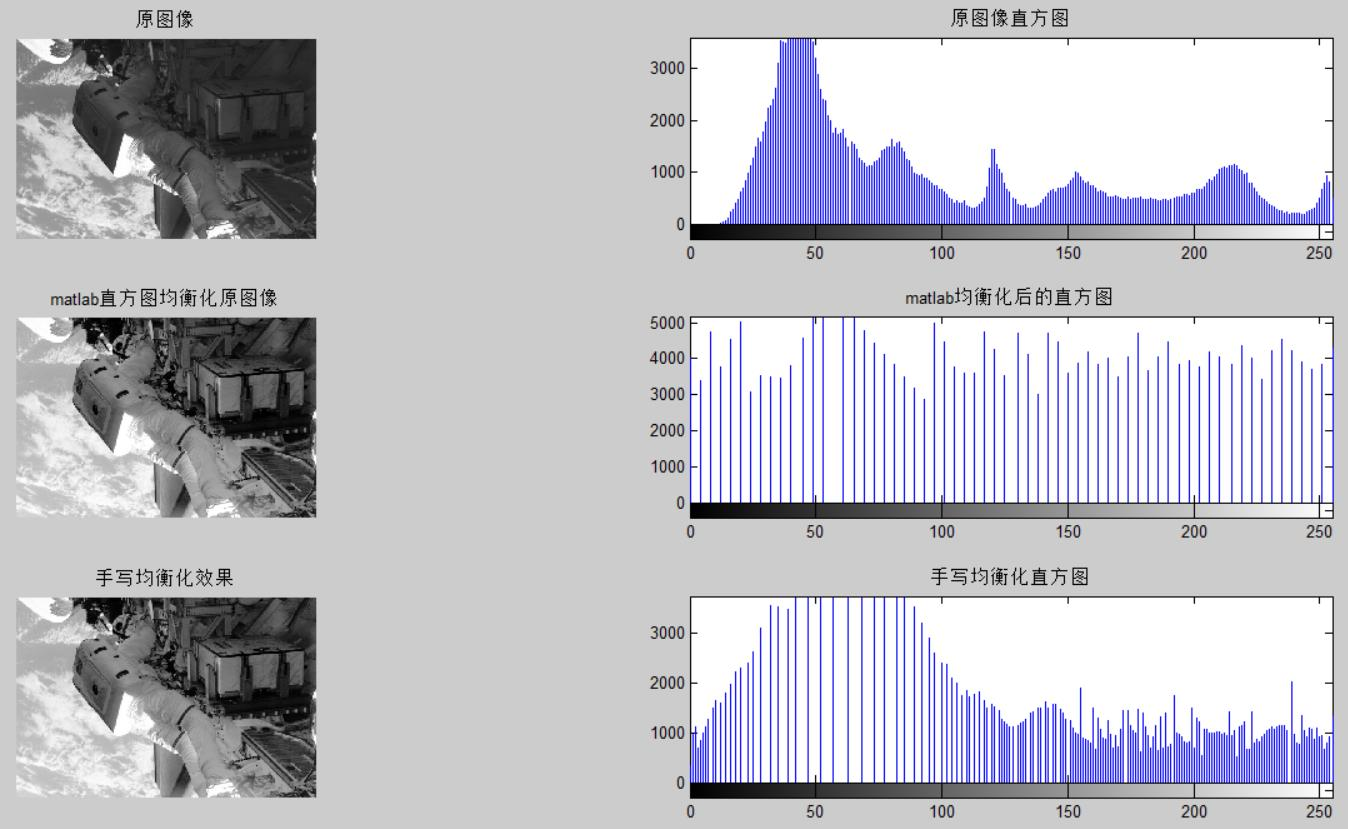为什们和matlab的直方图不一样呢？？？

二，指数变换
指数变换（Power-Law ）的公式：S=c*R^r,通过合理的选择c和r可以压缩灰度范围，算法以c=1.0/255.0, r=2实现。要做该图像增强变换需要先做归一化，再指数变换，最后反归一化
增强效果展示：可以看见，改增强算法并不能很好的将像素尽可能的碾平。指数增强参考程序为：

[cpp] view
plain copy

print?

clc;
close all;
clear all;

% -------------Gamma Transformations-----------------
Gamma = 0.4;
g2 = myExpEnhance(f,Gamma);

figure();
subplot(221);  imshow(f);  xlabel('a).Original Image');
subplot(222),imhist(f),title('原图像直方图');%显示原始图像直方图
subplot(223);  imshow(g2);  xlabel('b).Gamma Transformations \gamma = 0.4');
subplot(224),imhist(g2),title('增强图像直方图');%显示原始图像直方图

指数增强核心函数为：

[cpp] view
plain copy

print?

function dst_img=myExpEnhance(src_img,Gamma)
src_img = mat2gray(src_img,[0 255]);%将图像矩阵A中介于amin和amax的数据归一化处理， 其余小于amin的元素都变为0， 大于amax的元素都变为1。
C = 1;
g2 = C*(src_img.^Gamma);
%反归一化
max=255;
min=0;
dst_img=uint8(g2*(max-min)+min);

三，对数变换

对数变换主要用于将图像的低灰度值部分扩展，将其高灰度值部分压缩，以达到强调图像低灰度部分的目的。变换方法由下式给出。这里的对数变换，底数为（v+1），实际计算的时候，需要用换底公式。其输入范围为归一化的【0-1】，其输出也为【0-1】。对于不同的底数，其对应的变换曲线如下图所示。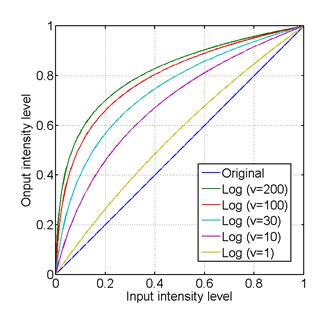底数越大，对低灰度部分的强调就越强，对高灰度部分的压缩也就越强。相反的，如果想强调高灰度部分，则用反对数函数就可以了。看下面的实验就可以很直观的理解，下图是某图像的二维傅里叶变换图像，其为了使其灰度部分较为明显，一般都会使用灰度变换处理一下。

效果图：参考代码：

[cpp] view
plain copy

print?

clc;
close all;
clear all;

%-------------Log Transformations-----------------

g_1 = myLogEnhance(f,10);
g_2 = myLogEnhance(f,100);
g_3 = myLogEnhance(f,200);

figure();
subplot(2,2,1);
imshow(f);xlabel('a).Original Image');

subplot(2,2,2);
imshow(g_1);xlabel('b).Log Transformations v=10');

subplot(2,2,3);
imshow(g_2);xlabel('c).Log Transformations v=100');

subplot(2,2,4);
imshow(g_3);
xlabel('d).Log Transformations v=200');

对数变换核心函数

[cpp] view
plain copy

print?

function dst_img=myLogEnhance(src_img,v)
c=1.0;
src_img = mat2gray(src_img,[0 255]);
g =c*log2(1 + v*src_img)/log2(v+1);
%反归一化
max=255;
min=0;
dst_img=uint8(g*(max-min)+min);

四，灰度拉伸

灰度拉伸也用于强调图像的某个部分，与伽马变换与对数变换不同的是，灰度拉升可以改善图像的动态范围。可以将原来低对比度的图像拉伸为高对比度图像。实现灰度拉升的方法很多，其中最简单的一种就是线性拉伸。而这里介绍的方法稍微复杂一些。灰度拉伸所用数学式如下所示。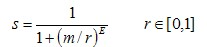同样的，其输入r为【0-1】，其输出s也为【0-1】。这个式子再熟悉不过了，跟巴特沃斯高通滤波器像极了，其输入输出关系也大致能猜到是个什么形状的。但是，这里就出现一个问题了，输入为0时候，式子无意义了。所以，在用Matlab计算的时候，将其变为如下形式。这里的eps，就是Matlab里面，一个很小数。如此做的话，式子变得有意义了。但是，其输入范围为【0-1】的时候，其输出范围变为了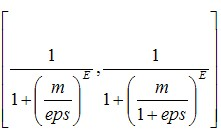。输出范围大致为【0-1】，为了精确起见，使用mat2gray函数将其归一化到精确的[0-1]。调用格式如下。

五，线性拉伸
为了突出感兴趣的目标或者灰度区间，相对抑制那些不感兴趣的灰度区域，可采用分段线性法，常用的是三段线性变换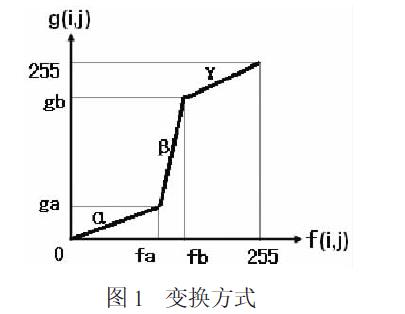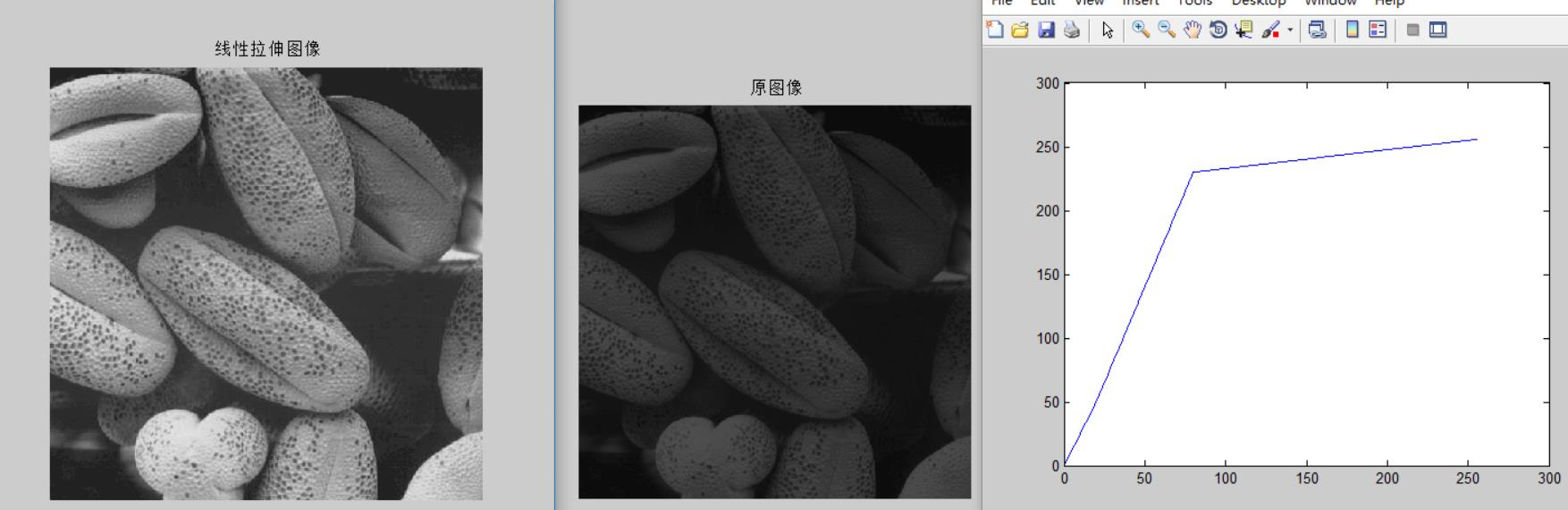参考程序：

[cpp] view
plain copy

print?

clc;
close all;
clear all;

[m,n,k]=size(I);
figure (1)
imshow('seed.tif');title(' 原图像');
mid=mean(mean(I));
%横轴
fa=20; fb=80;
%纵轴
ga=50; gb=230;

J=myLinearEnhance(I,fa,fb,ga,gb);
figure (2)
imshow(J);title(' 线性拉伸图像');

pixel_f=1:256;
pixel_g=zeros(1,256);

%三段斜率,小于1表示该段将会被收缩
k1=double(ga/fa);
k2=(gb- ga)/(fb- fa);
k3=(256- gb)/(256- fb);
for i=1:256
if i <= fa
pixel_g(i)= k1*i;
elseif fa < i && i <= fb
pixel_g(i)= k2*( i- fa)+ ga;
else
pixel_g(i)= k3*( i - fb)+ gb;
end
end
figure (3)
plot(pixel_f,pixel_g);

核心函数：

[cpp] view
plain copy

print?

function dst_img=myLinearEnhance(src_img,fa,fb,ga,gb)

[height,width] = size(src_img);
dst_img=uint8(zeros(height,width));

src_img=double(src_img);

%三段斜率
k1=ga/fa;
k2=(gb- ga)/(fb- fa);
k3=(255- gb)/(255- fb);
for i=1:height
for j=1:width
if src_img(i,j) <= fa
dst_img(i,j)= k1*src_img(i,j);
elseif fa < src_img(i,j) && src_img(i,j) <= fb
dst_img(i,j)= k2*( src_img(i,j)- fa)+ ga;
else
dst_img(i,j)= k3*( src_img(i,j)- fb)+ gb;
end
end
end
dst_img=uint8(dst_img);

附录：

附录网上的另一份讲解：
直方图均衡化算法分为三个步骤，第一步是统计直方图每个灰度级出现的次数，第二步是累计归一化的直方图，第三步是计算新的像素值。
第一步：
for(i=0;i<height;i++)
for(j=0;j<width;j++)
n[s[i][j]]++;

for(i=0;i<L;i++)
p[i]=n[i]/(width*height);

这里，n[i]表示的是灰度级为i的像素的个数，L表示的是最大灰度级，width和height分别表示的是原始图像的宽度和高度，所以，p[i]表示的就是灰度级为i的像素在整幅图像中出现的概率(其实就是p[]这个数组存储的就是这幅图像的归一化之后的直方图)。
第二步：
for(i=0;i<=L;i++)
for(j=0;j<=i;j++)
c[i]+=p[j];

c[]这个数组存储的就是累计的归一化直方图。
第三步：
max=min=s;
for(i=0;i<height;i++)
for(j=0;j<width;j++)
if(max<s[i][j]){
max=s[i][j];
}else if(min>s[i][j]){
min=s[i][j];
}

找出像素的最大值和最小值。
for(i=0;i<height;i++)
for(j=0;j<width;j++)
t[i][j]=c[s[i][j]]*(max-min)+min;

t[][]就是最终直方图均衡化之后的结果。

收录优秀代码：

这份代码写得不错，学习了，原博客地址见参考资源【3】！

[cpp] view
plain copy

print?

#include <stdio.h>
#include <iostream>
#include "fftw3.h"
#include "string"
#include "vector"
#include <windows.h>
#include <opencv2/legacy/legacy.hpp>
#include <opencv2/nonfree/nonfree.hpp>//opencv_nonfree模块：包含一些拥有专利的算法，如SIFT、SURF函数源码。
#include "opencv2/core/core.hpp"
#include "opencv2/features2d/features2d.hpp"
#include "opencv2/highgui/highgui.hpp"
#include <opencv2/nonfree/features2d.hpp>

using namespace cv;
using namespace std;

class hisEqt
{
public:
hisEqt::hisEqt();
hisEqt::~hisEqt();
public:
int w;
int h;
int nlen;

int *pHis;
float *pdf;

//=====求像素分布概率密度====
void  getPdf();

//======统计像素个数=======
void getHis(unsigned char*imgdata);

//==========画统计分布直方图===============
void drawHistogram(const float*pdf,Mat &hist1);

//===========直方图均衡化==========
void hisBal();

//====直方图均衡化后的图像===
void imgBal(unsigned char* img);
};

hisEqt::hisEqt() :nlen(0){
pHis = new int[256 * sizeof(int)];
memset(pHis, 0, 256 * sizeof(int));
pdf = new float[255 * sizeof(float)];
memset(pdf, 0, 255 * sizeof(float));
}

hisEqt::~hisEqt(){
delete[]pHis;
delete[]pdf;
}

//======统计像素个数=======
void hisEqt::getHis(unsigned char*imgdata){
for (int i = 0; i<nlen; i++)
{
pHis[imgdata[i]]++;
}
}

//=====求像素分布概率密度====
void hisEqt::getPdf(){
for (int k = 0; k<256; k++)
{
pdf[k] = pHis[k] / float(nlen);
}
}

//===========直方图均衡化==========
void hisEqt::hisBal(){
for (int k = 1; k<256; k++)
{
pdf[k] += pdf[k - 1];
}
for (int k = 0; k<256; k++)
{
pHis[k] = 255 * pdf[k];
}
}

//====直方图均衡化
void hisEqt::imgBal(unsigned char* img){
for (int i = 0; i<nlen; i++)
{
img[i] = pHis[img[i]];
}
}

void hisEqt::drawHistogram(const float *pdf, Mat& hist1){
for (int k = 0; k<256; k++)
{
if (k % 2 == 0)
{
Point a(k, 255), b(k, 255 - pdf[k] * 2550);
line(hist1,
a,
b,
Scalar(0, 0, 255),
1);
}
else
{
Point a(k, 255), b(k, 255 - pdf[k] * 2550);
line(hist1,
a,
b,
Scalar(0, 255, 0),
1);
}
}
}

int main()
{
if (!image.data)
return -1;

Mat hist2(256, 256, CV_8UC3, Scalar(0, 0, 0));
Mat hist1(256, 256, CV_8UC3, Scalar(0, 0, 0));

Mat imgOut = Mat(image.rows, image.cols, CV_8UC3, Scalar(0, 0, 0));
vector<Mat> planes;
int chn = image.channels();
if (chn == 3)
{
split(image, planes);
}
while (chn)
{
chn--;
unsigned char* imageData = new unsigned char[sizeof(unsigned char)*(image.cols*image.rows)];
memcpy(imageData, planes[chn].data, planes[chn].cols*planes[chn].rows);
hisEqt his;//自定义的类
his.nlen = image.rows*image.cols;
his.getHis(imageData);
his.getPdf();

//  //======画原图直方图并保存============
his.drawHistogram(his.pdf, hist1);
string pic_name = "hisline";
pic_name = pic_name + to_string(chn);
pic_name=pic_name+  ".jpg";
imwrite(pic_name, hist1);

his.hisBal();
his.getPdf();
//  //======画均衡化后直方图并保存============
his.drawHistogram(his.pdf, hist2);
string pic_name0 = "his_balanceline";
pic_name0 = pic_name0 + to_string(chn);
pic_name0 = pic_name0 + ".jpg";
imwrite(pic_name0, hist2);

//  //=====图像均衡化===
his.imgBal(imageData);
memcpy(planes[chn].data, imageData, planes[chn].cols*planes[chn].rows);
delete[] imageData;
imageData = NULL;
}
merge(planes, imgOut);//单通道合并
imwrite("result.jpg", imgOut);
return 0;
}


展开全文图像处理
• 对比度增强 提前规定：输入图像
对比度增强

提前规定：输入图像为?，宽为?，高为?，输出图像?，??,?为输入图像?的 ?行?列像素灰度级，??,?为输出图像?的?行?列像素灰度级，0≤?≤?,0≤?≤?

灰度直方图

灰度直方图描述了一幅图像中每个灰度级在图像中占据的像素个数或者是比率，利用灰度直方图，通过数学方法，我们可以很容易的实现图像的处理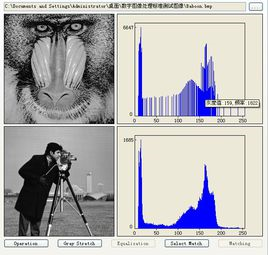1.1线性变换

输入图像为?,宽为?，高为?,输出图像? ,图像的线性变换可以表示为

??,?=?∗??,?+?,0≤?≤?,0≤?≤?

优点：简单易懂

缺点：通过该方法可以调整图像的对比度，但是参数?,?需要根据自己实际图像进行多次测试来确定，比较繁琐

1.2直方图正则化

直方图正则化，依据了统计学原理，来进行图像的对比度调整：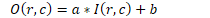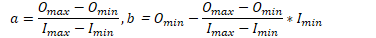一般规定????=255；????=0

优点：直方图正规化自动选取?,?的线性变换

缺点：1.变换后图像的灰度级减少，某些细节消失；2.某些图像，如直方图有高峰，经处理后对比度不自然的过分增强

1.3 伽马变换

伽马变换的方法，首先将灰度值诡异到[0.1]范围，??,?表示归一化之后的像素值

??,?=??,??，0≤?≤?,0≤?≤?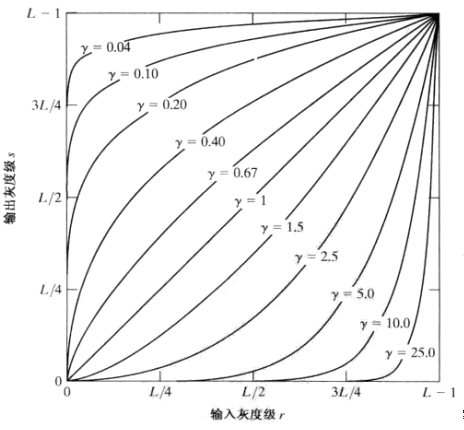输出图像不变：伽马=1

输入图像整体过暗，可以调整令 0<γ<1, 来增加对比度

输入图像整体过暗,  可以调整令   γ>1对比度

优点：提升对比度效果比较好

缺点：需要手动调整γ值

1.4 全局直方图均衡

原图 ? 的直方图是各个像素级分布不均匀，导师对比度出现问题，全局直方图均衡使得输出图像?的像素均匀分布在每一像素级别，输出图像?的对比度达到一个比较好的效果。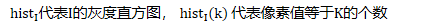全局直方图均衡就是在做这个工作，找出亮度级别为p的输入像素到亮度级别为q的输出像素的映射，最终使得输出图像?的像素均匀分布在每一像素级别。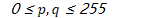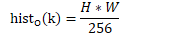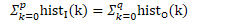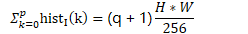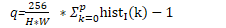所以推得：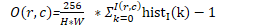1.5限制对比度的自适应直方图均衡化

将图形划分为不重叠的区域，单独对每个区域做直方图均衡化。为了避免每个区域中的噪声被放大，使用限制对比度的方法，某点像素值超过了该区域的限制对比度，则将起均匀分配给该区域中其他的像素点。

展开全文• ## 图像对比度增强算法

万次阅读 多人点赞 2017-04-24 09:11:45图像处理 对比度增强
• 本章主要总结了图像增强技术中的点处理技术，包括灰度直方图的获取、直方图均衡化、直方图规范化和对比度调整等内容。C++和Python语言环境下，使用OpenCV工具实现上述图像增强操作。 OpenCV 简介： 1、OpenCV的...图像增强 OpenCV
• 对比度拉伸是图像增强的一种方法，也属于灰度变换操作。我们看如下图像： 可以看到，这张图片非常灰暗。我们查看下其直方图。 import cv2 import matplotlib.pyplot as plt farina = cv2.imread("far...
• 1）改善图像的视觉效果，2）转换为更适合于人或机器分析处理的形式3）突出对人或机器分析有意义的信息4）抑制无用信息，提高图像的使用价值5）增强后的图像并不一定保真2，图像增强的方法分类：1）从处理对象分类：...
• 最近在制作一个安卓上的图像处理软件，感觉调整对比度这个功能，在photoshop上还是比较常用的功能，于是就在软件上加上这个功能。 对比度的定义：简单的来说就是使亮的地方更亮，暗的地方更暗。 但是关于亮与...图像处理
• 通过使用matlab将图片的对比度提升。程序如下：% 通过灰度直方图的数据显示该图像的灰度值整体偏高，图像过于明亮， % 所以选用 γ &gt; 1 的伽马变换 % 降低图像的亮度，提升图片的对比度。 clc img1 = imread...数字图像处理 matlab
• ## 图像增强之对比度拉伸

万次阅读 多人点赞 2018-03-24 13:39:59
对比度拉伸是图像增强的一种方法，也属于灰度变换操作。我们看如下图像： 可以看到，这张图片非常灰暗。我们查看下其直方图。 import cv2 import matplotlib.pyplot as plt farina = cv2.imread(...
• 点运算又称为对比度增强对比度拉伸或灰度变换，是一种通过图像中的每一个像素值进行运算的图像处理方式。。它将输入图像映射为输出图像，输出图像每个像素点的灰度值仅有对应的输入像素点的灰度值决定，运算结果...MATLAB imadjust 灰度变换 图像处理
•   提到图像增强，第一印象就是直方图均衡与直方图规定化，这是最常见的也是非常有效的全局图像增强方法。在前不久的一次组会讨论中，课题组的一位同学提到了“自适应图像增强”，虽然自己以前也用过，但是一时间...自适应对比度增强 对比度增强
• 图像增强的方法分类：1 从处理...邻域方法，即空域滤波），频域方法4 从处理目的分类：图像锐化，平滑去噪，灰度调整（对比度增强）二 图像增强的方法之对比度增强1 灰度变换法线性变换（已实现）对数变换（已实...
• ## 图像处理之调整亮度与对比度

万次阅读 多人点赞 2012-03-22 22:57:57
图像处理之调整亮度与对比度   很多时候，一张图像被过度曝光显得很白，或者光线不足显得很暗，有时候背景跟图像人物 也观察不清楚，这个时候可以通过调节图像的两个基本属性-亮度与对比度来获得整体效果 的...图像处理 float 算法 filter algorithm
• 目录   饱和度 色调 对比度 ...图像处理(image processing)，用计算机对图像进行分析，以达到所需结果的技术。...图像处理技术的一般包括图像压缩，增强和复原，匹配、描述和识别3个部分。 常见的系统有康...
• 其实在图像处理中，拉开对比度是最重要的一项。常用的拉开对比度方式和使用算子如下： 1.将RGB图像拆分通道 算子：decompose3()将RGB图像拆分成三个通道 R G B，数字3表示按照3通道拆分 -> trans_from_...计算机视觉
• 图像对比度增强 1、图像增强处理并不能增加原始图像的信息，只能增强对某种信息的辨别能力，而这种处理肯定会损失一些其它信息； 2、强调根据具体应用而言，更“好”更“有用”的视觉效果图像； 3、图像增强处理...对比度增强
• 小波变换下的图像对比度增强技术实质上是通过小波变换把图像信号分解成不同子带，针对不同子带应用不同的算法来增强不同频率范围内的图像分量，突出不同尺度下的近似和细节，从而达到增强图像层次感的目的。...开发人员 图像处理 机器学习 小波变换 图像增强
...